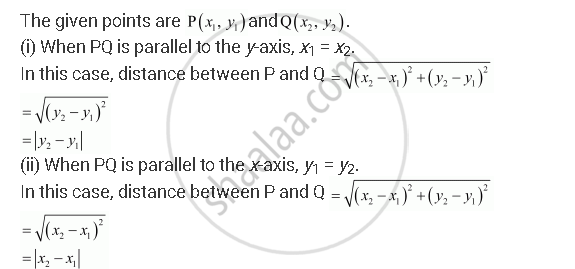CBSE (Arts) Class 11CBSE
Share

# Find the Distance Between P (X1, Y1) and Q (X2, Y2) When : (I) Pq is Parallel to the Y-axis, (Ii) Pq is Parallel to the X-axis - CBSE (Arts) Class 11 - Mathematics

#### Question

Find the distance between P (x1, y1) and Q (x2, y2) when : (i) PQ is parallel to the y-axis, (ii) PQ is parallel to the x-axis

#### SolutionIs there an error in this question or solution?

#### Video TutorialsVIEW ALL 

Solution Find the Distance Between P (X1, Y1) and Q (X2, Y2) When : (I) Pq is Parallel to the Y-axis, (Ii) Pq is Parallel to the X-axis Concept: Slope of a Line.
S